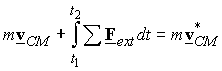The linear impulse-momentum relation

Linear momentum of a particle: The symbol L denotes the linear momentum and is defined as the mass times the velocity of a particle.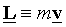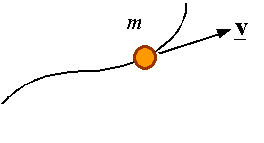Impulse of a force from time t1 to t2: The integral of the force over the time interval of concern is its impulse. The impulse of a force is a vector given by the integral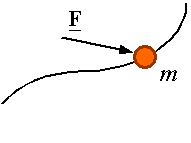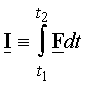Newton’s 2nd law: Newton’s second law states that the resultant of all forces applied on a particle is equal to the rate of change of linear momentum of the particle.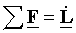This reduces to the more familiar statement of this law if one notes that in Newtonian mechanics it is assumed that mass is constant, and, therefore,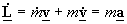.

The linear impulse-momentum relation: Integration of Newton’s 2nd law over the time interval from t1 to t2 results in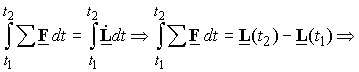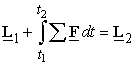Therefor, the linear momentum of a particle is changed by the impulse of the resultant force on the particle. There will be conservation of linear momentum only if the impulse of the resultant force is zero.

For a system of particles: Consider the system of particles shown below. Each particle in the system has a mass mi and at time t1 has a velocity vi, and from time t1 to time t2 is acted upon by the resultant external force Fi and the internal forces of interaction between the particles of fij.  As a result of the impulse of the internal and external forces on each particle, at time t2 the particle with mass mi has a velocity v*i.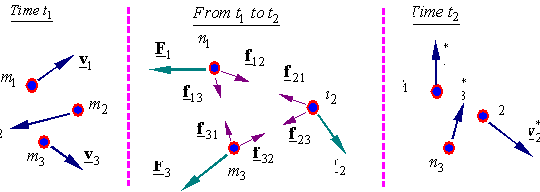Writing the impulse momentum equation for each particle and adding them up, considering that Newton’s 3rd law requires that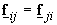, we get for n particles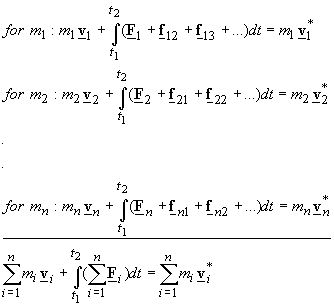Therefore, the total linear momentum of the system is changed by the impulse of the external forces. Recalling the relation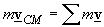, we can rewrite this equation as